# A Y = A+ B 1 1 1 1 1 1 Apply the idea of the truth table above and design a 3-bit comparator using XOR or XNOR gates of...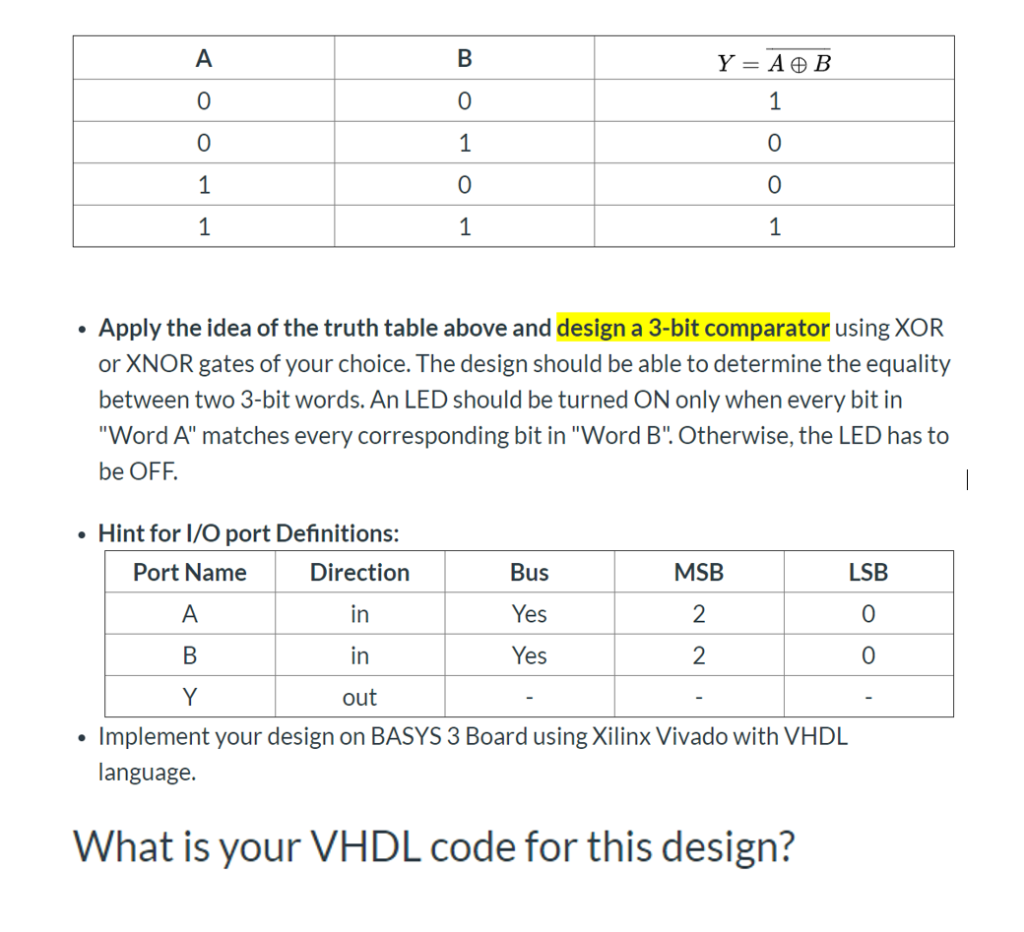A Y = A+ B 1 1 1 1 1 1 Apply the idea of the truth table above and design a 3-bit comparator using XOR or XNOR gates of your choice. The design should be able to determine the equality between two 3-bit words. An LED should be turned ON only when every bit in "Word A" matches every corresponding bit in "Word B". Otherwise, the LED has to be OFF Hint for I/O port Definitions: Port Name MSB Direction Bus LSB A in Yes 2 Yes 2 in out Implement your design on BASYS 3 Board using Xilinx Vivado with VHDL language. What is your VHDL code for this design?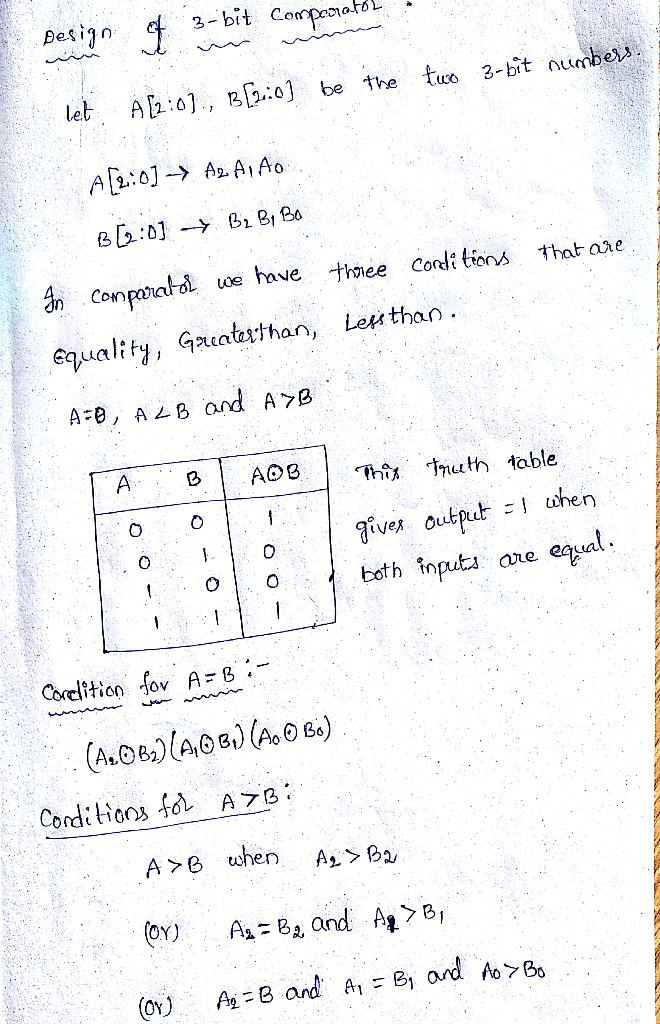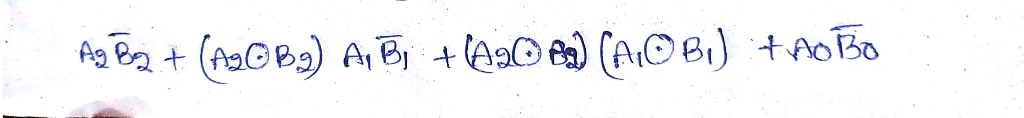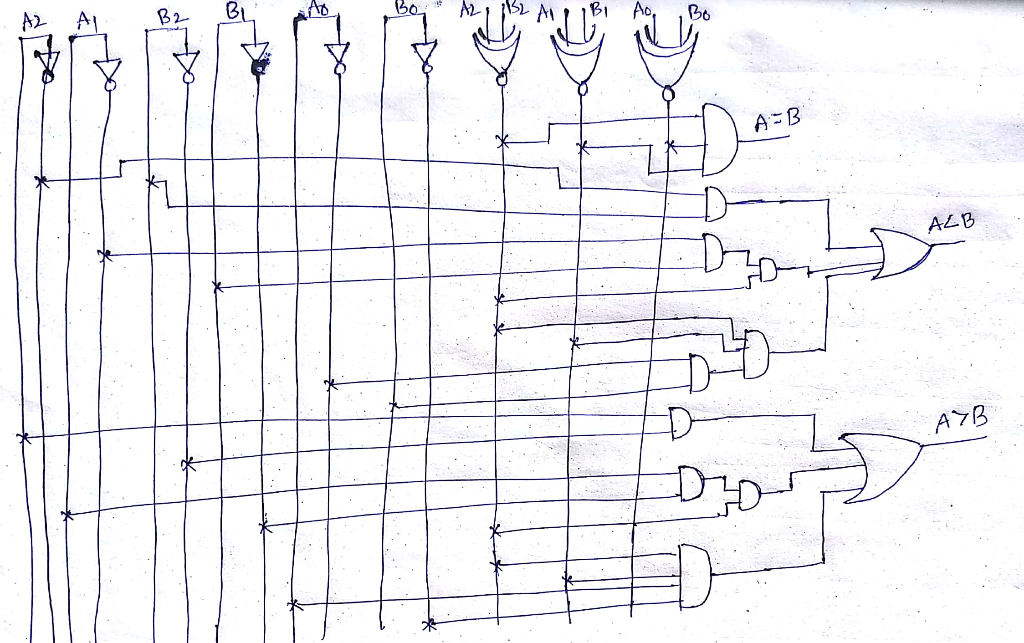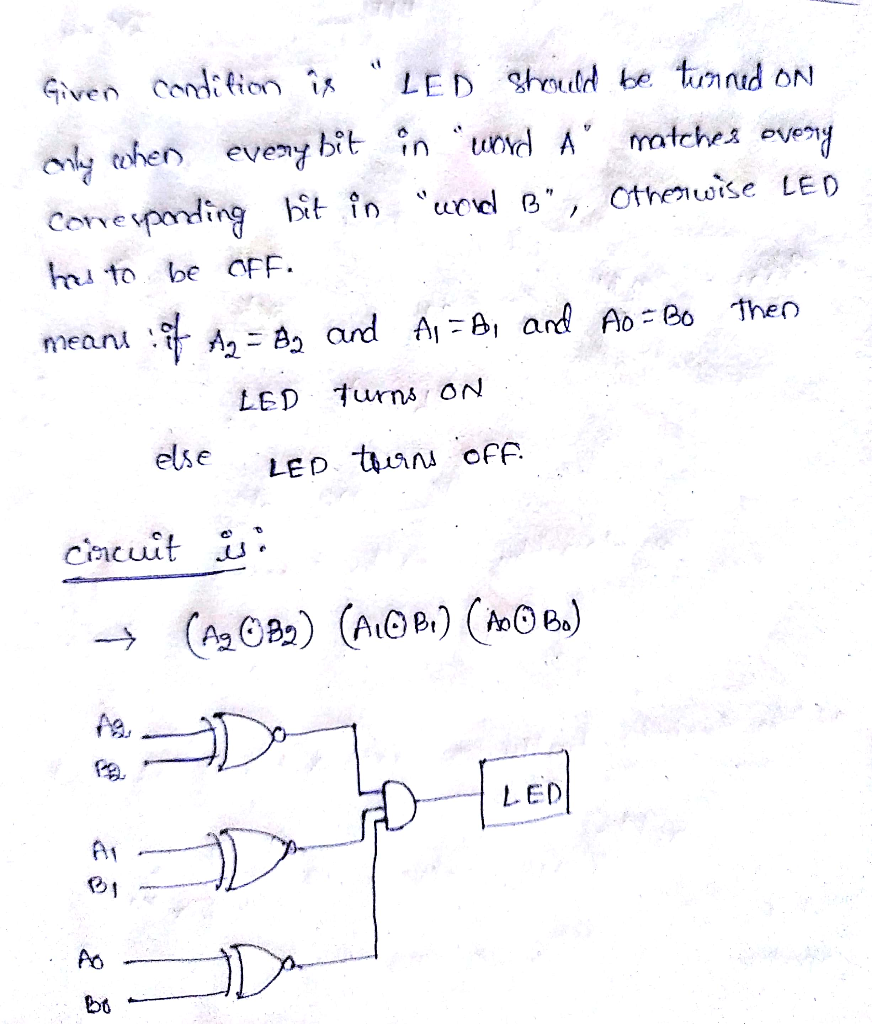##### Add Answer of: A Y = A+ B 1 1 1 1 1 1 Apply the idea of the truth table above and design a 3-bit comparator using XOR or XNOR gates of...
Similar Homework Help Questions
• ### A Y = A ® B 1 1 1 1 1 Apply the idea of the truth table above and design a 3-bit comparator using XOR or XNOR gates of...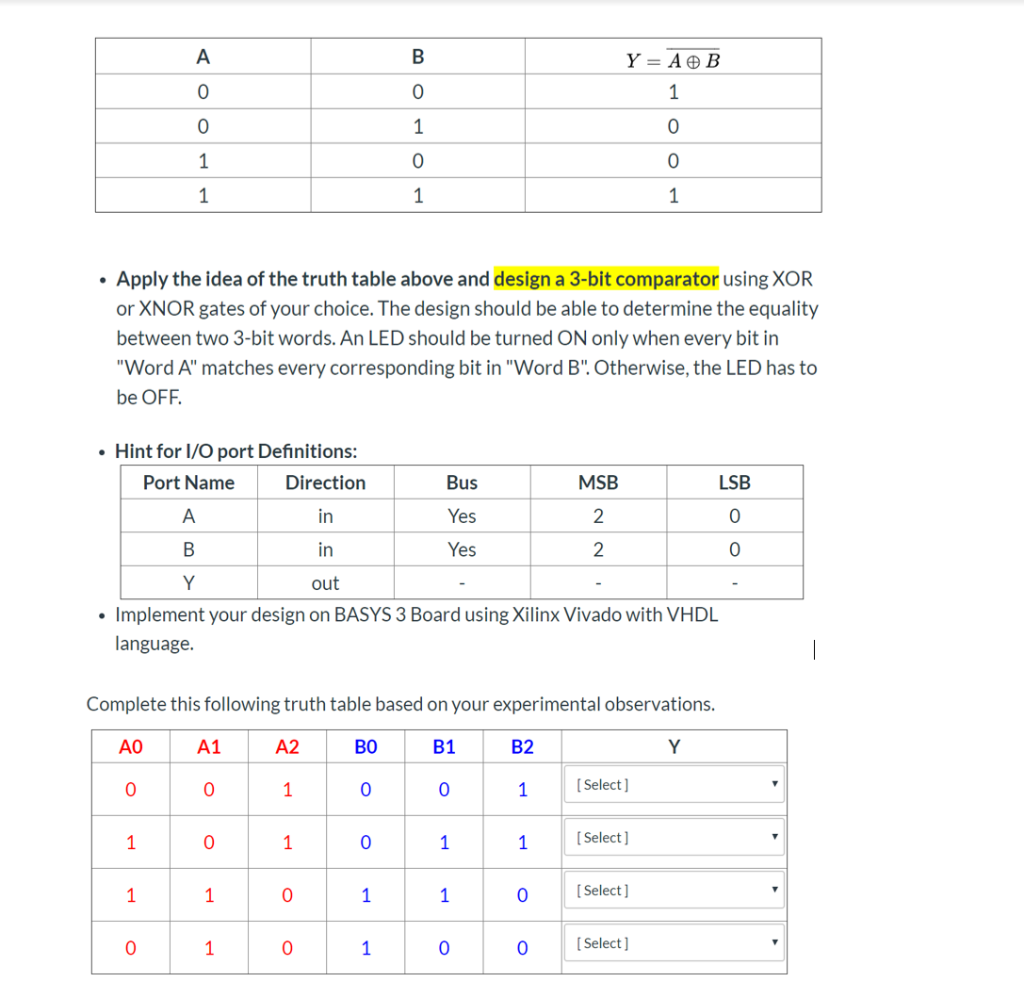A Y = A ® B 1 1 1 1 1 Apply the idea of the truth table above and design a 3-bit comparator using XOR or XNOR gates of your choice. The design should be able to determine the equality between two 3-bit words. An LED should be turned ON only when every bit in "Word A" matches every corresponding bit in "Word B". Otherwise, the LED has to be OFF Hint for I/O port Definitions: Port Name Direction...

• ### A Y = A ® B 0 1 1 1 0 1 1 1 Apply the idea of the truth table above and design a 3-bit comparator using XOR or XNOR gat...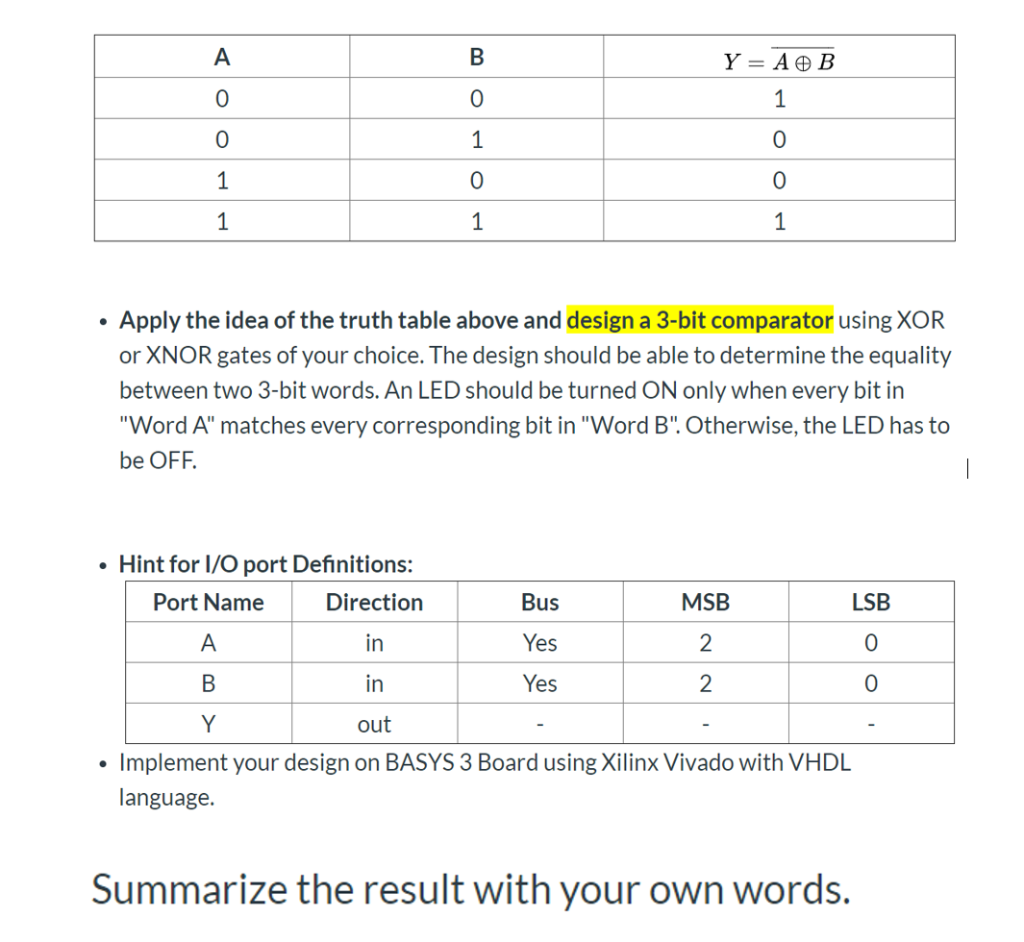A Y = A ® B 0 1 1 1 0 1 1 1 Apply the idea of the truth table above and design a 3-bit comparator using XOR or XNOR gates of your choice. The design should be able to determine the equality between two 3-bit words. An LED should be turned ON only when every bit in "Word A" matches every corresponding bit in "Word B".Otherwise, the LED has to be OFF Hint for I/O port Definitions: MSB...

• ### A Y = A ® B 1 1 1 0 0 1 1 1 Apply the idea of the truth table above and design a 3-bit comparator using XOR or XNOR gat...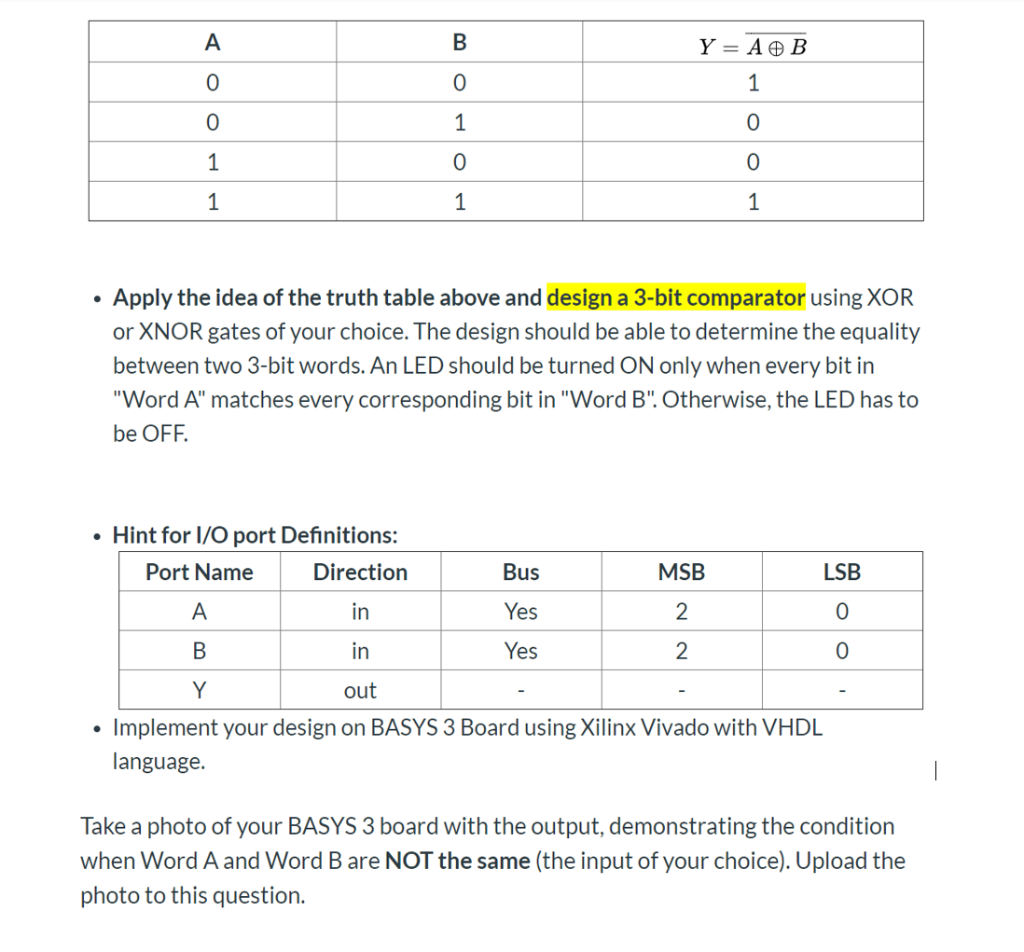A Y = A ® B 1 1 1 0 0 1 1 1 Apply the idea of the truth table above and design a 3-bit comparator using XOR or XNOR gates of your choice. The design should be able to determine the equality between two 3-bit words. An LED should be turned ON only when every bit in "Word A" matches every corresponding bit in "Word B". Otherwise, the LED has to be OFF. Hint for I/O port Definitions:...

• ### Use as few 3-input NOR gates as possible to design a bubble detector circuit for 8-bit thermomete...

Use as few 3-input NOR gates as possible to design a bubble detector circuit for 8-bit thermometer code. An n-bit thermometer code represents an integer m, with m 1s followed by (n-m) 0s. 1-bit bubble is an error in coding when a solitary 0 (or 1) is found in between two 1s (or 0s). What is the size of your circuit in terms of the number of NOR gates used? Give a gate level schematic diagram for your circuit. Implement...

• ### i. Design and test a 3-to-8 decoder with active-low outputs using VHDL/HDL.    Demonstrate your outputs in the BASYS board. (Note: Capture the pictures of your    output and add in in your answer scr...

i. Design and test a 3-to-8 decoder with active-low outputs using VHDL/HDL.    Demonstrate your outputs in the BASYS board. (Note: Capture the pictures of your    output and add in in your answer script)       ii. Include (screenshot) VHDL codes and .xdc file modification in your answer script.      iii. Develop a truth table following your outputs.

• ### (20 pts)VHDL. Implement the logic circuit specified in the following truth table by using a 4:1 m...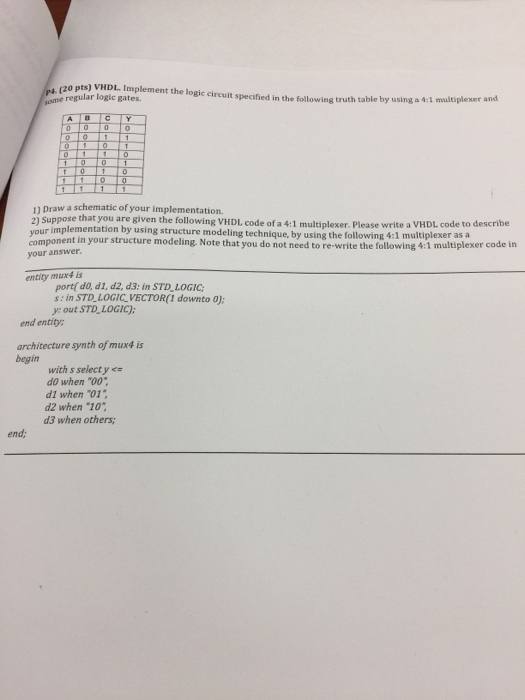(20 pts)VHDL. Implement the logic circuit specified in the following truth table by using a 4:1 mulitiplexer ome regular logic gates. 11 Draw a schematic of your implementation. 2) Suppose that you are given the following VHDL code of a 4:1 multiplexer. Please write a VHDL code to describe your implementation by using structure modeling technique, by using the following 4:1 multiplexer asia your answer component in your structure modeling. Note that you do not need to re-write the following...

• ### (h) Using the following relationship: and the relationship determined in (h), design a 1-bit adde...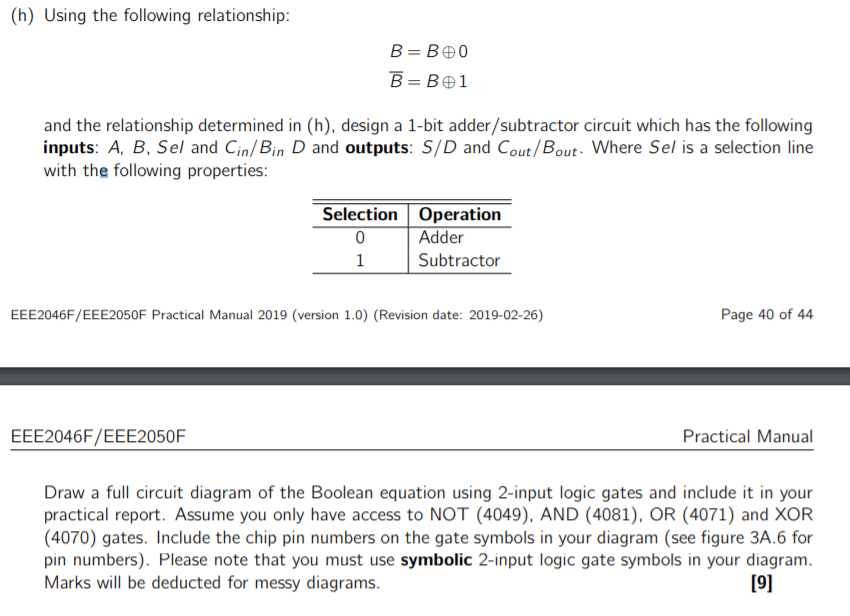(h) Using the following relationship: and the relationship determined in (h), design a 1-bit adder/subtractor circuit which has the following inputs: A, B, Sel and Cin/Bin D and outputs: S/D and Cout/Bout Where Sel is a selection line with the following properties Selection Operation 1 Subtractor Page 40 of 44 EEE2046F/EEE2050F Practical Manual 2019 (version 1.0) (Revision date: 2019-02-26) EEE2046F/EEE2050F Practical Manual Draw a full circuit diagram of the Boolean equation using 2-input logic gates and include it in your...

• ### The circuit below takes as input a four bit unsigned binary number A A2 A Ao and generates a single output F. Design th...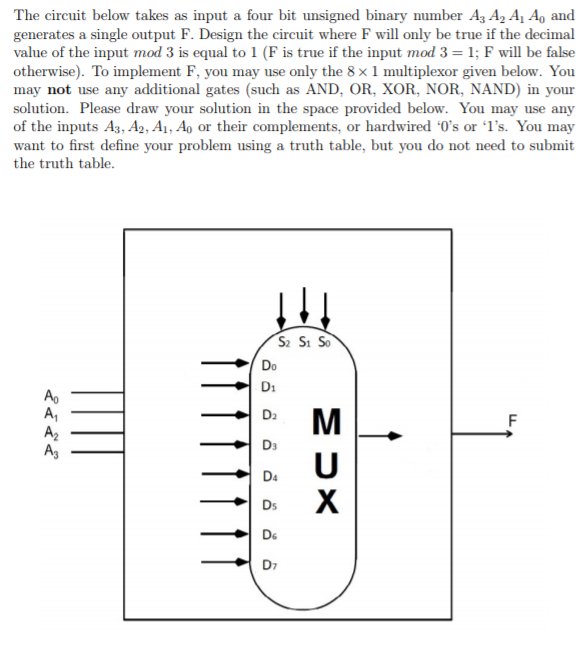The circuit below takes as input a four bit unsigned binary number A A2 A Ao and generates a single output F. Design the circuit where F will only be true if the decimal value of the input mod 3 is equal to 1 (F is true if the input mod 3- 1; F will be false otherwise). To implement F, you may use only the 8 x 1 multiplexor given below. You may not use any additional gates (such...

• ### Student ID K-map to simply the function f e and "d" is the least si (3 points each) CO: 3] 3. Five bits of information and a parity bit are to be transmitted on a noisy channel. The trans...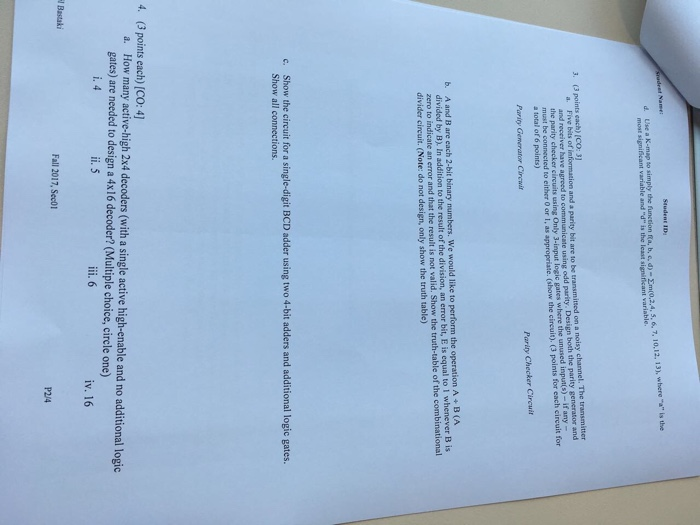Student ID K-map to simply the function f e and "d" is the least si (3 points each) CO: 3] 3. Five bits of information and a parity bit are to be transmitted on a noisy channel. The transmittor a. the parity checker circuits using Only 3-imput logic gates where the unused inpunts)-if any- must be connected to either O or 1, as appropriate. (show the cireuit). (3 points for each circuit for a total of 6 points) ver have...

• ### Q2 (20pts) Design a combinational ct that accepts an input 3-bit binary number (XYZ) and generate...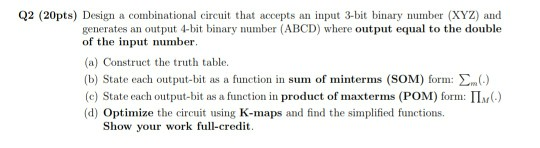Q2 (20pts) Design a combinational ct that accepts an input 3-bit binary number (XYZ) and generates an output 4-bit binary number (ABCD) where output equal to the double of the input number. (a) Construct the truth table (b) State each output-bit as a function in sum of minterms (SOM) form: (c) State each output-bit as a function in product of maxterms (POM) form: ΠΜ(.) (d) Optimize the circuit using K-maps and find the simplified functions Show your work full-credit. Q3...

Need Online Homework Help?